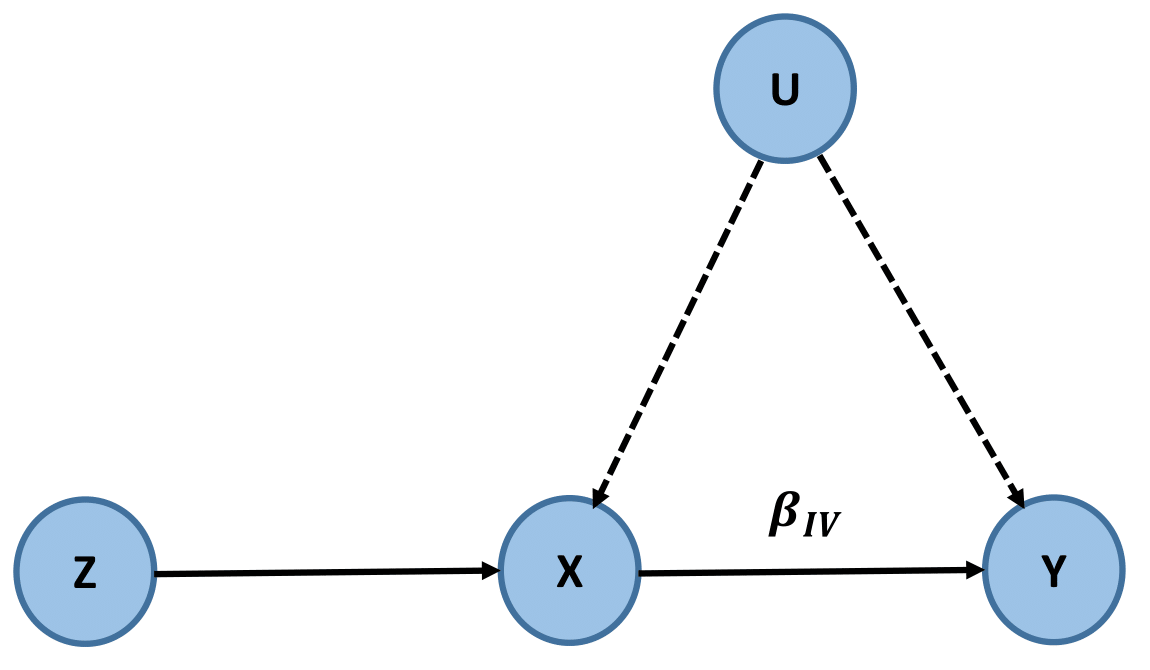# Instrumental Variable

Published:

## Preliminary

• Sample Mean $\mu_X$ :

\begin{align} \mu_X = \frac{1}{N}\sum^N_{i=1}x_i \label{eq1}\tag{1} \end{align}
• Standard deviation $\sigma_X$ :

\begin{align} \sigma_X = \sqrt{\bf{E}[ {(X-\mu_x)}^2 ]} \\= \sqrt{\frac{1}{N} \sum^N_{i=1}(x_i-\mu_X)^{2} } \label{eq2}\tag{2} \end{align}
• Covariance $COV_{XY}$:
\begin{align} COV_{XY} = \bf{E}[ {(X-\mu_X) (Y-\mu_Y) } ] \label{eq3}\tag{3} \end{align}
• Correlation $r_{XY}$ :
\begin{align} r_{XY} = \frac{COV_{XY}}{\sigma_X\sigma_Y} =\frac{\bf{E}[ {(X-\mu_X) (Y-\mu_Y) } ]} {\sigma_X\sigma_Y} \\ = \frac{\sum^N_{i=1}(x_i-\mu_X)(y_i-\mu_Y)} { N \sigma_X\sigma_Y }\\=\frac{\sum^N_{i=1}(x_i-\mu_X)(y_i-\mu_Y)} { \sqrt{ \sum^N_{i=1}(x_i-\mu_X)^{2} \sum^N_{i=1}(y_i-\mu_Y)^{2} } } \label{eq4}\tag{4} \end{align}
• Slope of OLS $\min_{\beta_0,\beta_1} \sum^N_{i=1}(y_i-\beta_0 - \beta_1x_i)^2$ :

\begin{align} \beta_1 = \frac{dy}{dx}= \frac{\sum^N_{i=1} (x_i-\mu_X) (y_i-\mu_Y) }{\sum^N_{i=1} (x_i -\mu_X)^2 } \label{eq5}\tag{5} \end{align}

## Instrumental Variable## Definition of IV:

• The IV $Z$ is only associated with the exposure $X$.
• The IV $Z$ is not associated with the unobserved confounder $U$ of the exposure $X$ and the outcome $Y$.

## Estimation of $\beta_{IV}$:

\begin{align} y=\beta_{IV}x+\mu \label{eq6}\tag{6} \end{align}

Multiply $\frac{1}{z}$ for both sides of (\ref{eq6})

We get

\begin{align} \frac{y}{z}= \beta_{IV} \frac{x}{z} +\frac{\mu}{z} \label{eq7}\tag{7} \end{align}

If we calculate the derivative with respective to $z$, we have

\begin{align} \frac{dy}{dz}= \beta_{IV} \frac{dx}{dz} +\frac{d\mu}{dz} \label{eq8}\tag{8} \end{align}

Since $\mu$ is uncorrelated with $z$, we have the following estimate

\begin{align} \beta_{IV} = \frac{dy/dz}{dx/dz} \label{eq9}\tag{9} \end{align}

Plugin (\ref{eq5}), (\ref{eq4}), and (\ref{eq3}) into (\ref{eq9}), we have

\begin{align} \beta_{IV} &= \frac{dy/dz}{dx/dz} \\ &= \frac{\sum^N_{i=1} (z_i-\mu_Z) (y_i-\mu_Y) }{\sum^N_{i=1} (z_i -\mu_Z)^2 } \frac{\sum^N_{i=1} (z_i -\mu_Z)^2}{\sum^N_{i=1} (z_i-\mu_Z) (x_i-\mu_X)} \\ &= \frac{\sum^N_{i=1} (z_i-\mu_Z) (y_i-\mu_Y) }{\sum^N_{i=1} (z_i-\mu_Z) (x_i-\mu_X) } \\&= \frac{COV_{ZY}}{COV_{ZX}} \\&= \frac{r_{ZY}\sigma_Y} {r_{ZX}\sigma_X} \label{eq10}\tag{10} \end{align}

### Interpretation of $\beta_{IV}$ :

• The change in the $Y$ for a unit change in the $X$ is equal to the change in $Y$ for a unit change of $Z$ scaled by the change in $X$ for a unit change of $Z$.